Market

The Kelly CriterionHow to apply the celebrated bet-sizing and CASH-management formula in trading and wagering.

Gamblers and traders alike should get to know the Kelly criterion intimately.The formula, developed in 1956 by Bell Labs scientist John Kelly, uses Information Theory to calculate how much to wager or invest to maximize long-term wealth.

But the criterion is often poorly understood, and its misuse leads to the ruin of many would-be traders. Their wealth skyrockets and then collapses to zero because they fail to grasp that the line between defeat and long-term profit is written in the language of mathematics.

Two keys unlock success in professional gambling and serious trading:

Identifying profitable opportunities. Correctly sizing bets. No. 1 is the easy part. No. 2 separates the professionals from the amateurs.

Trend-following “turtle” trader Michael W. Covel put it this way: Trading correctly is 90% money management and portfolio management.

A trader with a mediocre strategy and a great model for risk and bet-sizing will become fairly successful. A trader with a great strategy and a mediocre risk model will go bankrupt.

Examples abound. Think of a blackjack player deciding what percentage of his bankroll to wager on a given hand, a real estate investor who’s determining how much of her portfolio to commit to a new property or a cryptocurrency trader deciding what leverage to apply to a new strategy.

Expected Value

Imagine a betting opportunity that offers positive expected value (EV) with known payouts and probabilities. A blackjack player, for example, knows that the current running count and true count imply a win/loss probability for the next hand of 52% versus 48%.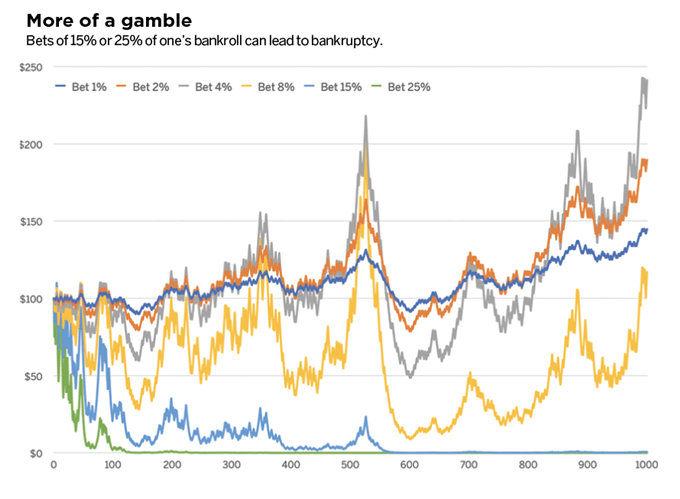A 52% chance of winning seems attractive, but how much of the gambler’s total net worth should he wager on it?

Deciding that requires balancing the competing forces of betting more to achieve greater profit and betting less to limit the chance of going broke.

Somewhere between those extremes there’s an optimal proportion of the total bankroll to bet such that long-term wealth is maximized.

Size Matters

An uncomplicated experiment can test what happens when a card player makes bets of differing sizes on more than 1,000 hands of blackjack.

In one example, the gambler wagers 1% of his bankroll on each hand, plotting the course of his net worth over 1,000 simulated hands. The strategy makes a nice return over time, with a bit of volatility.

After 1,000 hands, the gambler has increased his wealth by 44% (see Bet 1% line in “More of a gamble,” above).

But what if a gambler risks a larger proportion of his net worth on each bet? The chart above shows his profit over time with bet sizes of 1%, 2% and 4% of net worth per hand.

It’s not surprising that as the size of the bet increases, the profit and the variance of the gambler’s net worth does likewise. Cumulative winnings grow steadily as the bet size increases from 1% to 4%.

Yet, with larger bets, something counter-intuitive creeps into the simulation. Take for example the following bets: 1%, 2%, 4%, 8%, 15% and 25%.

Bets of 8% can slow the growth of the gambler’s bankroll. Bets of 15% or 25% can lead to bankruptcy.

In fact, a gambler who bets too much is losing money with winning bets. Larger and larger wagers continually increase the variance of his net worth, but beyond a point, profits peak and then reverse.

But why would doing more of a good thing result in a worse long-term outcome?

Negative geometric drag

To understand this rise and fall in returns, imagine an especially good gambling opportunity: A bet with 50/50 probability and a payout of 2:1 (\$2 gain for \$1 wagered).

Clearly, that’s a profitable opportunity, but how much should a gambler invest in each round of the bet?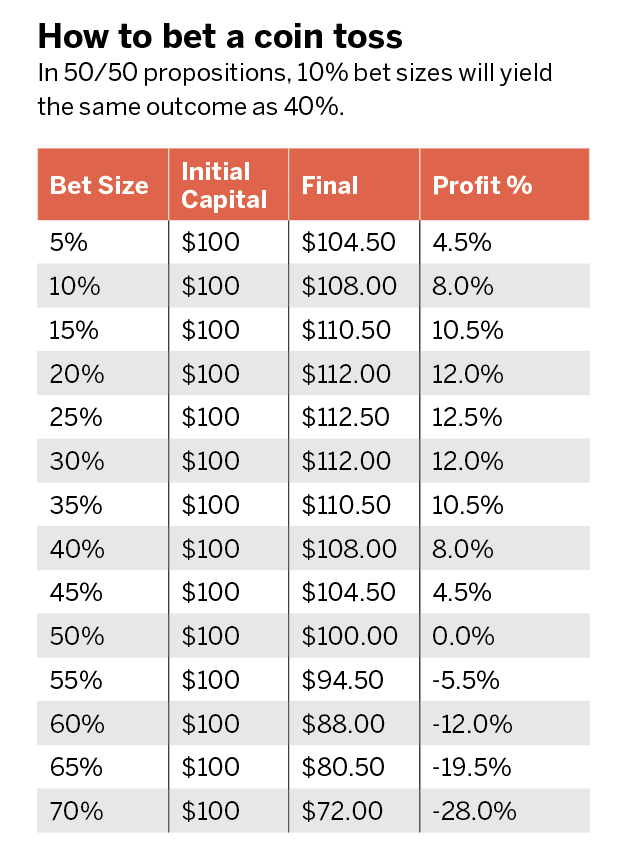In the first example, 1,000 rounds of betting were simulated.

This time, a more mathematical approach can help. Because the bet wins or loses with equal probability (50/50), an average round of betting produces an equal number of wins and losses. The simplest model of bets would be one win and one loss, and the order doesn’t matter.

As bet size (left column in “How to bet a coin toss,” left) increases, the size of the wins and losses increases, too. The total profit (right column) increases with bet size but then plateaus and falls.

A wager of 40% per round is as profitable as 10% per round. Yet, 60% actually loses money on average. But why?

The losses are caused by negative geometric drag (NGD).

This drag on a portfolio’s profit results arithmetically from gaining and losing the same proportion of value. A gain of X% followed by a loss of X% (or a loss followed by a gain of the same amount), always results in a net loss.

A loss of 10% on an investment followed by a 10% gain results in having 0.99 of the original investment (0.90 x 1.10 = 0.99) or a 1% net loss. Profiting 20% then losing 20% nets a 4% loss from the starting point (1.20 x 0.8 = 0.96). The drag on an investment’s return is the square of the gain or loss.

Edge + NGD = profit

As leverage is increased, the edge of a bet grows linearly with the amount of leverage, but the NGD grows as the square of the leverage.

At lower levels of leverage, the edge is the dominant force and the NGD is negligible. However, as leverage grows, the NGD becomes larger and eventually overwhelms the betting edge.

Shown graphically in “Kelly curve,” below, these figures create the familiar Kelly curve (blue). Profit (red) is the sum of the ever-increasing leverage and the negative geometric drag (gray).

The green vertical line indicates where the two countervailing forces perfectly offset each other (marginal edge = marginal NGD). This is the point of maximum profit (bet size = 0.25), and any additional leverage would result in lower profit.

To state this another way, on 50/50 outcomes, using this betting size (0.25 of net worth) would maximize the geometric growth rate wealth over time.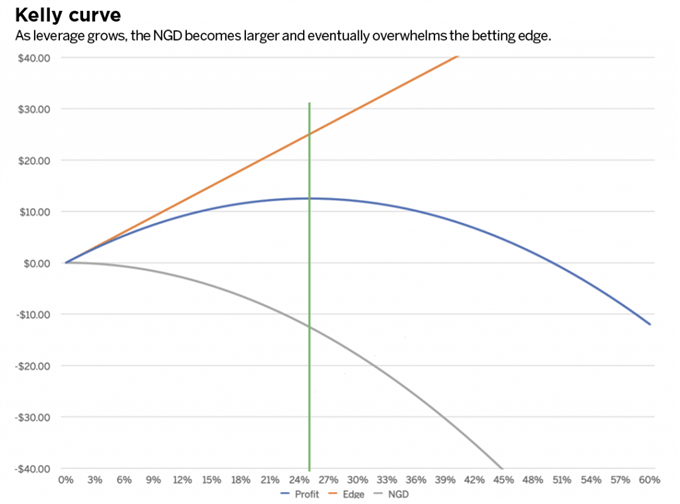Putting Kelly to work

The Kelly criterion formalizes this logic in a single formula. It accepts known probabilities and payoffs as inputs and returns the proportion of total wealth to bet for maximum growth.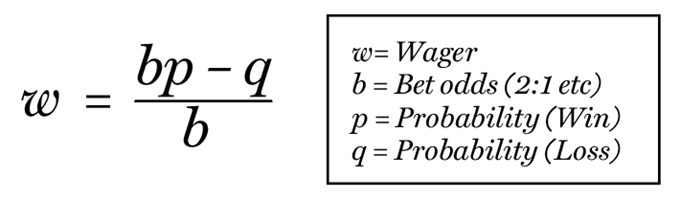The example above would set b=2, p=0.5 and q=0.5. Wager size solves to 0.25, exactly the same value as the trial-and-error table above.

What about a startup investment or ICO that has a 15% chance of success but a 30x payoff? A question like this is tough to work out intuitively, but the Kelly criterion advises an investment of 12% of total capital.

Returning to the card counter from the introduction, b=1 p=0.52 q=0.48.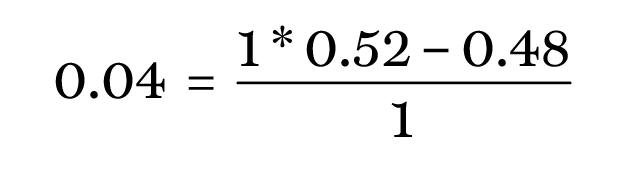The gambler should bet 4% of his bankroll—exactly as predicted. But how do these principles hold up in the real world? A look at Ethereum (ETH) trading can help answer this.

Kelly on Crypto

Ethereum trading offers an excellent real-world application of Kelly. A particular ETH long volatility/trend-following strategy has the following returns from 2016 to the present in which the Yaxis is annualized return and the X-axis is leverage.

The long volatility strategy has a maximum (Kelly optimal) return of 644% per year versus 225% for holding long ETH (2016 to present).

This optimal return used 1.7x leverage. Leverage of 1x, 2x and 4x would have returned +418%, +602% and -99% (total loss) annually. By using too much or too little leverage, the trader can easily miss out on an opportunity or even lose everything.

The Kelly-optimal bet is a fine line based on a solid understanding of the probabilities and returns.

Kelly ≠ Goal

Kelly = Limit

The Kelly criterion only defines the “optimal” bet to maximize return. It does not use caution or assign value to risk.

Kelly represents the limit to the range of rational investments. It is simply the largest bet that could still be rational assuming no value is placed on risk.

Betting even one penny more than Kelly would bring increased risk, increased variance and decreased profit.

However, bet-sizing anywhere near the Kelly-optimal amount is irrational by most standards.

As the bet size approaches the Kelly-optimal point, the ratio of additional risk to additional profit goes to infinity. Eventually, the investor would have to risk an additional \$1 billion dollars to earn one cent more of expected profit.

Most people assign a negative value to risk. That’s why we pay a premium to insurance companies to haul away excess risk.

As a result, professional traders use leverage that is partway up the Kelly curve.

Kelly is not the goal but rather the boundary. The ETH strategy had a Kelly optimal leverage of 1.7x, yielding 644% annualized return. But a leverage of just 1.3x returned 520% annual profit with only half of the variance.

The Sharpe ratio (Return/Variance) also improves with lower fractions of Kelly. In fact, the more modest leverage of 1.3x improves the Sharpe of the ETH strategy from 1.90 to 3.04. As a rule-of-thumb, many traders use “half-Kelly” which offers 75% of the maximum profit with just 25% of the variance. This is a 3x better risk-adjusted return.

As Ed Seykota, a pioneer of systems trading, once said, “There are old traders, and there are bold traders. There are very few old, bold traders.”

The Kelly criterion is an excellent tool for assessing the qualitative shape of risk versus reward and understanding the boundaries of rational bets. While it does not assign value to risk, simple heuristics like ‘half-Kelly’ are enough for real-world application.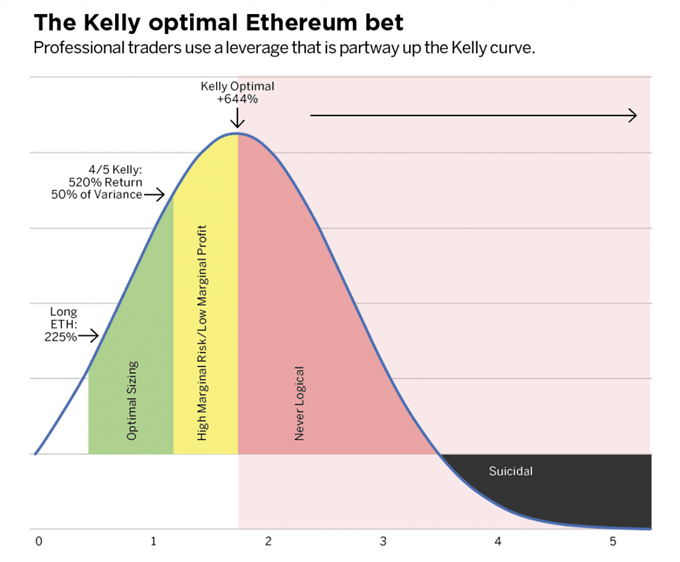Nicholas Yoder, a hedge fund manager and former firefighter, studied mathematics and machine learning at Carnegie Mellon University. @nickyoder86.